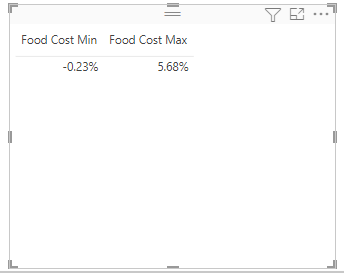cancel
Showing results for
Did you mean:Helper III

## MinX and MaxX on a Measure while filtering for a Category

Hello everyone and thank you in Advance. I am still fairly new to DAX and starting to get the grasp of it. I am still having a problem trying to use the Min and Max commands (I tried MIN, MINA, MINX, etc) when applying the forumla on a measure. I believe I need to use MINX and MAXX since it refers to a measure. I might need to make these measure in the table I am referencing the measure too (I had to do this for RANKX to work) but Im honestly not sure as I have tried that as well. Here is my syntax:

Food Cost Max = IF([Cost %]>0, MAXX(FILTER(ChartOfAcounts, [Category] = "Food Cost"), All_Actual_Expenses[Cost %]))

For context, Cost % is a measure that divides Revenues by Expenses. We assigned Category Numbers to each expense and Revenue. The categories are outlined in a different table.

So basically I am trying to create Mins and Maxs for Food Cost, Labor cost, and Gross Cost to be able to set up an 'Outliers' Group to put into a different visual. The idea is for this visualization to consistly show outliers.

However, I cant get there unless I fix the Max and Min formulas. I am expecting to see the Min be 30.85% and the Max be 37.37% (I have circled these in the image below).Here are results with the above formula1 ACCEPTED SOLUTIONSuper User

@james_pease

``````Food Cost Max =
MAXX (
CALCULATETABLE (
VALUES ( ChartOfAcounts[Store_ID] ),
'ChartOfAccounts'[Category] = "Food Cost"
),
CALCULATE ( [Cost %], 'ChartOfAccounts'[Category] = "Food Cost" )
)``````
8 REPLIES 8Helper III

Also, I am using a data slicer to filter the 5 companies separetly. So I am looking to pull the outliers for each company separetly. So I will be making 5 separate visualizations or 1 visualizations but have the min and max be categorized by each company. I think that is a simple filter(company name that I can add to the mins and maxs formulas.Super User

@james_pease
Yes should not be an issue. Have you tried my proposed formula?Helper III

Category is a Column from a table named 'ChartOfAccounts'Super User

@james_pease

``````Food Cost Max =
MAXX (
CALCULATETABLE (
VALUES ( ChartOfAcounts[Store_ID] ),
'ChartOfAccounts'[Category] = "Food Cost"
),
CALCULATE ( [Cost %], 'ChartOfAccounts'[Category] = "Food Cost" )
)``````Helper III

Thank you so much! I only had to modify the Store ID section (we have stores in a different table) but worked like a charm! Thanks again! Now I can replicate the formula for the other cost measures and add a filter clause to ensure each company is being calculated separately.Helper III

Sorry, should have clarified, yes each dot represents a store. So we graphed them with cost % on the y axis and revenue on the x axis. I know that it should be flipped as revenus is the dependant variable but thats how the company wanted it graphed.Super User

@james_pease
Is [Category] a column or a measure?Super User

what does each dot represent? A store?